# 一篇详尽的HashMap整理（面试看这一篇就够了）1 HashMap总结

2 HashMap底层实现

2.1 AbstractMap类

2.2 重要内部类 / 接口

2.2.1 Entry接口和Node类

2.2.2 KeySet 类

2.2.3 Values类

2.2.4 EntrySet类

2.3 HashMap 1.7 底层结构

2.4 HashMap 1.8 底层结构

2.4.1 jdk1.8 的其他改进

2.5 HashMap 重要属性

2.5.1 初始容量

2.5.2 最大容量

2.5.3 默认负载因子

2.5.4 树化阈值

2.5.5 链表阈值

2.5.6 扩容临界值

2.5.7 扩容阈值

2.6 HashMap构造函数

2.7 put全过程

2.8 扩容机制

2.9 get全过程

3 HashMap面试细节

3.1 两个对象的 hashCode 相同会发生什么？

3.2 你知道 hash 的实现吗？为什么要这样实现？

3.3 为什么要用异或运算符？

3.4 为什么要保证 capacity 是2的次幂呢？

3.5 capacity 都是 2 次幂，如果我们指定 initialCapacity 不为 2次幂时，是不是就破坏了这个规则？

3.6 链表法导致链表过深问题为什么不用二叉查找树代替，而选择红黑树？为什么不一直使用红黑树？

3.8 HashMap & TreeMap & LinkedHashMap 使用场景？

3.9 HashMap 和 HashTable 有什么区别？

3.10 ava 中的另一个线程安全的与 HashMap 极其类似的类是什么？同样是线程安全，它与 HashTable 在线程同步上有什么不同？

3.11 针对 ConcurrentHashMap 锁机制具体分析（JDK 1.7 VS JDK 1.8）？

3.12 ConcurrentHashMap 在 JDK 1.8 中，为什么要使用内置锁 synchronized 来代替重入锁 ReentrantLock？

3.13 ConcurrentHashMap 的并发度是什么？

3.14 ConcurrentHashMap 简单介绍

HashMap 是 Map 接口的实现，允许key的值为null，但是是一个非线程安全的容器，如果想构造线程安全的 Map 使用 ConcurrentHashMap。 也可以用

`` Collections.synchronizedMap(new HashMap) ``

HashMap 无法保证内部存储的键值对的有序性所以是无序的。

HashMap 的底层数据结构是数组（又称桶） + 链表。遍历 HashMap 需要的时间损耗由桶的数量 和 键值对数量决定。因此，如果遍历元素很重要的话，不要把初始容量设置的太高或者负载因子设置的太低。

HashMap 有两个重要参数：初始容量、负载因子，初始容量指的就是 hash 表桶的数量，负载因子是一种衡量哈希表填充程度的标准，当哈希表中存在足够数量的键值对时，哈希表会进行扩容。

HashMap 尽量要用迭代器自己的 remove 方法，外部 remove 方法都可能会导致 fail-fast 机制。如果在迭代器创建的过程中修改了 map 的结构，会抛出异常

``ConcurrentModificationException ``

HashMap的继承 / 实现结构如下：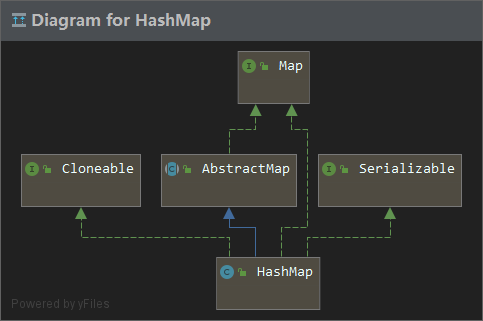## 2.1 AbstractMap类

AbstractMap抽象类实现了一些简单且通用的方法，为了实现不可修改的 map，仅需要继承这个类并且提供 entrySet 方法的实现即可。它将会返回一组 map 映射的某一段，返回的集合是 Set 类型，不应该支持 add 或者 remove 方法，并且它的迭代器也不支持 remove 方法。

## 2.2 重要内部类 / 接口

### 2.2.1 Entry接口和Node类

Map中定义了内部接口Entry，如下：

map的entry就是一个键值对

``````interface Entry<K,V> {
K getKey();
V getValue();
V setValue(V value);
boolean equals(Object o);
int hashCode();
}``````

HashMap中定义了静态内部类？Node 实现了Map中的Entry接口，Node 节点会存储四个属性，hash值，key，value，指向下一个Node节点的引用，并且也要实现上述五个方法：

``````final int hash;
final K key;
V value;
Node<K,V> next;``````

### 2.2.2 KeySet 类

KeySet 类继承于 AbstractSet 抽象类，是由 HashMap 中的 keySet()方法来创建实例的，旨在对HashMap 中的键进行操作。

KeySet() 在 Map 接口中进行定义的，在 HashMap 中将其操作进行了实现。

``````// 返回一个Set集合，包含了map中的key。
public Set<K> keySet() {
// 这里 keySet 是 AbstractMap 中的
Set<K> ks = keySet;
if (ks == null) {
// 如果 ks 为空，就创建一个 KeySet 对象
ks = new KeySet();
keySet = ks;
}
return ks;
}``````

``````final class KeySet extends AbstractSet<K> {
public final int size()                 { return size; }
public final void clear()               { HashMap.this.clear(); }
public final Iterator<K> iterator()     { return new KeyIterator(); }
public final boolean contains(Object o) { return containsKey(o); }
public final boolean remove(Object key) {
return removeNode(hash(key), key, null, false, true) != null;
}
public final Spliterator<K> spliterator() {
return new KeySpliterator<>(HashMap.this, 0, -1, 0, 0);
}
public final void forEach(Consumer<? super K> action) {
Node<K,V>[] tab;
if (action == null)
throw new NullPointerException();
if (size > 0 && (tab = table) != null) {
int mc = modCount;
for (int i = 0; i < tab.length; ++i) {
for (Node<K,V> e = tab[i]; e != null; e = e.next)
action.accept(e.key);
}
if (modCount != mc)
throw new ConcurrentModificationException();
}
}
}``````

### 2.2.3 Values类

``````public Collection<V> values() {
Collection<V> vs = values;
if (vs == null) {
vs = new Values();
values = vs;
}
return vs;
}``````

``````final class Values extends AbstractCollection<V> {
public final int size()                 { return size; }
public final void clear()               { HashMap.this.clear(); }
public final Iterator<V> iterator()     { return new ValueIterator(); }
public final boolean contains(Object o) { return containsValue(o); }
public final Spliterator<V> spliterator() {
return new ValueSpliterator<>(HashMap.this, 0, -1, 0, 0);
}
public final void forEach(Consumer<? super V> action) {
Node<K,V>[] tab;
if (action == null)
throw new NullPointerException();
if (size > 0 && (tab = table) != null) {
int mc = modCount;
for (int i = 0; i < tab.length; ++i) {
for (Node<K,V> e = tab[i]; e != null; e = e.next)
action.accept(e.value);
}
if (modCount != mc)
throw new ConcurrentModificationException();
}
}
}``````

### 2.2.4 EntrySet类

HashMap中还有直接对键值对进行操作的内部类 EntrySet，同样的是由 entrySet() 方法进行创建的，也是HashMap实现的Map接口的方法：

``````// 返回一个 Set 集合，此集合包含了 map 中的键值对
public Set<Map.Entry<K,V>> entrySet() {
Set<Map.Entry<K,V>> es;
// 如果 es 为空创建一个新的 EntrySet 实例
return (es = entrySet) == null ? (entrySet = new EntrySet()) : es;
}``````

``````final class EntrySet extends AbstractSet<Map.Entry<K,V>> {
public final int size()                 { return size; }
public final void clear()               { HashMap.this.clear(); }
public final Iterator<Map.Entry<K,V>> iterator() {
return new EntryIterator();
}
public final boolean contains(Object o) {
if (!(o instanceof Map.Entry))
return false;
Map.Entry<?,?> e = (Map.Entry<?,?>) o;
Object key = e.getKey();
Node<K,V> candidate = getNode(hash(key), key);
return candidate != null && candidate.equals(e);
}
public final boolean remove(Object o) {
if (o instanceof Map.Entry) {
Map.Entry<?,?> e = (Map.Entry<?,?>) o;
Object key = e.getKey();
Object value = e.getValue();
return removeNode(hash(key), key, value, true, true) != null;
}
return false;
}
public final Spliterator<Map.Entry<K,V>> spliterator() {
return new EntrySpliterator<>(HashMap.this, 0, -1, 0, 0);
}
public final void forEach(Consumer<? super Map.Entry<K,V>> action) {
Node<K,V>[] tab;
if (action == null)
throw new NullPointerException();
if (size > 0 && (tab = table) != null) {
int mc = modCount;
for (int i = 0; i < tab.length; ++i) {
for (Node<K,V> e = tab[i]; e != null; e = e.next)
action.accept(e);
}
if (modCount != mc)
throw new ConcurrentModificationException();
}
}
}``````

## 2.3 HashMap 1.7 底层结构

JDK1.7 中，HashMap 采用数组 + 链表的实现，链表被用来处理冲突。当位于一个桶中的元素较多时，即 hash 值相等的元素较多时，通过 key 依次查找的效率较低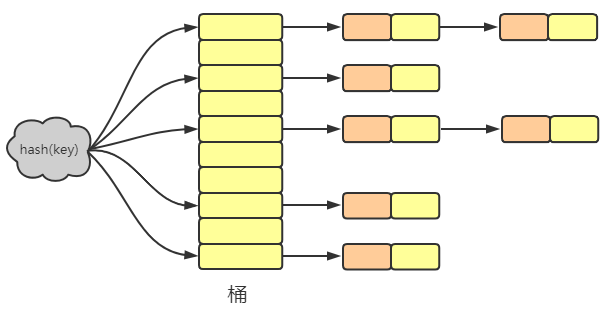HashMap的桶其实就是一个 Node 数组，链表的每一个节点都是 Node 的一个具体对象，存储了hash，key，value，next属性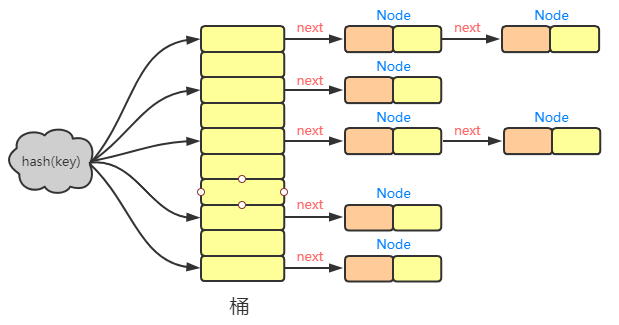## 2.4 HashMap 1.8 底层结构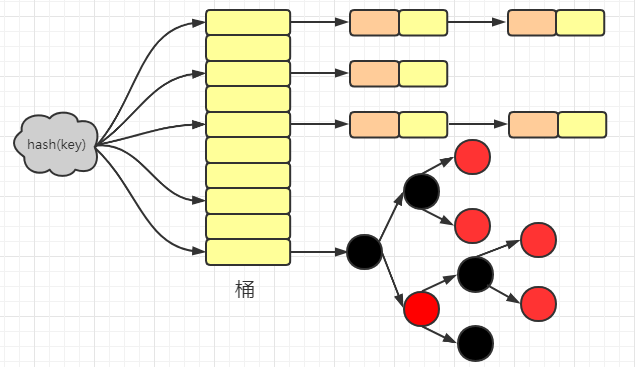### 2.4.1 jdk1.8 的其他改进

• 优化hash()方法

• 扩容时插入顺序的改进
• 其他
- 函数方法：forEach, compute系列 - Map的新API：merge, replace

## 2.5 HashMap 重要属性

### 2.5.1 初始容量

HashMap 的默认初始容量是由 DEFAULT_INITIAL_CAPACITY 属性确定的。默认值是16。

``static final int DEFAULT_INITIAL_CAPACITY = 1 << 4;``

### 2.5.2 最大容量

``static final int MAXIMUM_CAPACITY = 1 << 30;``

### 2.5.3 默认负载因子

``static final float DEFAULT_LOAD_FACTOR = 0.75f;``

HashMap 中存储的数量 > HashMap 容量 * 负载因子

### 2.5.4 树化阈值

``static final int TREEIFY_THRESHOLD = 8;``

### 2.5.5 链表阈值

``static final int UNTREEIFY_THRESHOLD = 6;``

### 2.5.6 扩容临界值

``static final int MIN_TREEIFY_CAPACITY = 64;``

### 2.5.7 扩容阈值

``````static final int tableSizeFor(int cap) {
int n = cap - 1;
n |= n >>> 1;
n |= n >>> 2;
n |= n >>> 4;
n |= n >>> 8;
n |= n >>> 16;
return (n < 0) ? 1 : (n >= MAXIMUM_CAPACITY) ? MAXIMUM_CAPACITY : n + 1;
}``````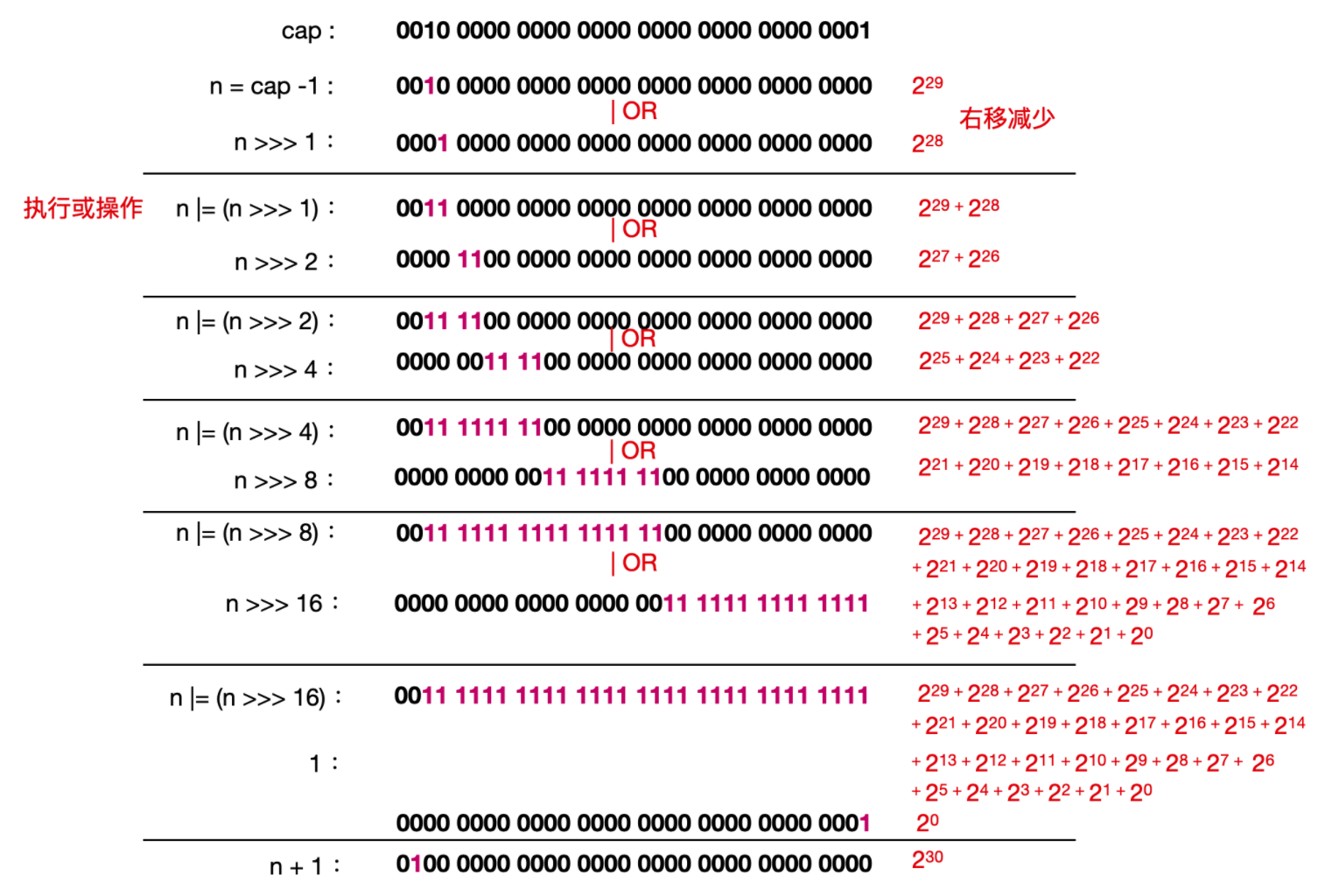## 2.6 HashMap构造函数

• 传入初始容量 initialCapacity 和负载因子 loadFactor 的构造方法
``````public HashMap(int initialCapacity, float loadFactor) {
if (initialCapacity < 0)
throw new IllegalArgumentException("Illegal initial capacity: " +
initialCapacity);
if (initialCapacity > MAXIMUM_CAPACITY)
initialCapacity = MAXIMUM_CAPACITY;
throw new IllegalArgumentException("Illegal load factor: " +
this.threshold = tableSizeFor(initialCapacity);
}``````
• 只带有 initialCapacity 的构造函数
``````public HashMap(int initialCapacity) {
}``````

• 无参构造函数
``````public HashMap() {
}``````

• 带有map的构造函数
``````public HashMap(Map<? extends K, ? extends V> m) {
putMapEntries(m, false);
}``````

## 2.7 put全过程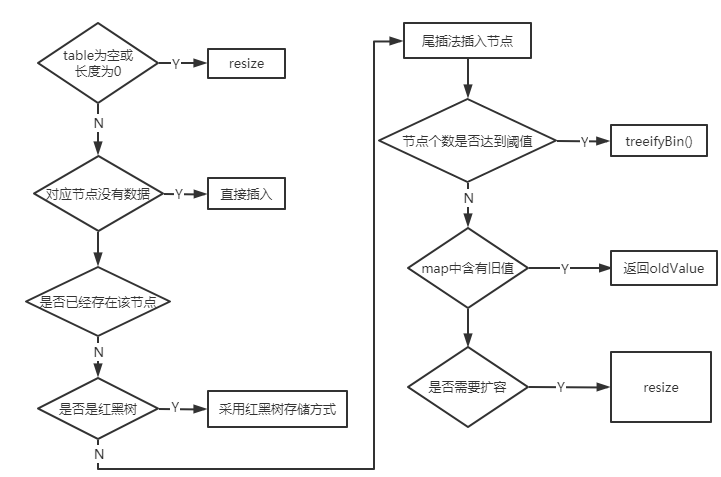具体的源代码如下，可以结合注释以及上面的示意图来看

``````final V putVal(int hash, K key, V value, boolean onlyIfAbsent,
boolean evict) {
Node<K,V>[] tab; Node<K,V> p; int n, i;
// 如果table 为null 或者没有为 table 分配内存，就resize一次
if ((tab = table) == null || (n = tab.length) == 0)
n = (tab = resize()).length;
// 指定hash值节点为空则直接插入，这个(n - 1) & hash才是表中真正的哈希
if ((p = tab[i = (n - 1) & hash]) == null)
tab[i] = newNode(hash, key, value, null);
// 如果不为空
else {
Node<K,V> e; K k;
// 计算表中的这个真正的哈希值与要插入的key.hash相比
if (p.hash == hash &&
((k = p.key) == key || (key != null && key.equals(k))))
e = p;
// 若不同的话，并且当前节点已经在 TreeNode 上了
else if (p instanceof TreeNode)
// 采用红黑树存储方式
e = ((TreeNode<K,V>)p).putTreeVal(this, tab, hash, key, value);
// key.hash 不同并且也不再 TreeNode 上，在链表上找到 p.next==null
else {
for (int binCount = 0; ; ++binCount) {
if ((e = p.next) == null) {
// 在表尾插入
p.next = newNode(hash, key, value, null);
// 新增节点后如果节点个数到达阈值，则进入 treeifyBin() 进行再次判断
if (binCount >= TREEIFY_THRESHOLD - 1) // -1 for 1st
treeifyBin(tab, hash);
break;
}
// 如果找到了同 hash、key 的节点，那么直接退出循环
if (e.hash == hash &&
((k = e.key) == key || (key != null && key.equals(k))))
break;
// 更新 p 指向下一节点
p = e;
}
}
// map中含有旧值，返回旧值
if (e != null) { // existing mapping for key
V oldValue = e.value;
if (!onlyIfAbsent || oldValue == null)
e.value = value;
afterNodeAccess(e);
return oldValue;
}
}
// map调整次数 + 1
++modCount;
// 键值对的数量达到阈值，需要扩容
if (++size > threshold)
resize();
afterNodeInsertion(evict);
return null;
}``````

• hash : put 放在桶中的位置，在 put 之前，会进行 hash 函数的计算。
• key : 参数的 key 值
• value : 参数的 value 值
• onlyIfAbsent : 是否改变已经存在的值，也就是是否进行 value 值的替换标志
• evict : 是否是刚创建 HashMap 的标志

## 2.8 扩容机制

HashMap 中的扩容机制是由 resize() 方法来实现的，程序流程图大致如下：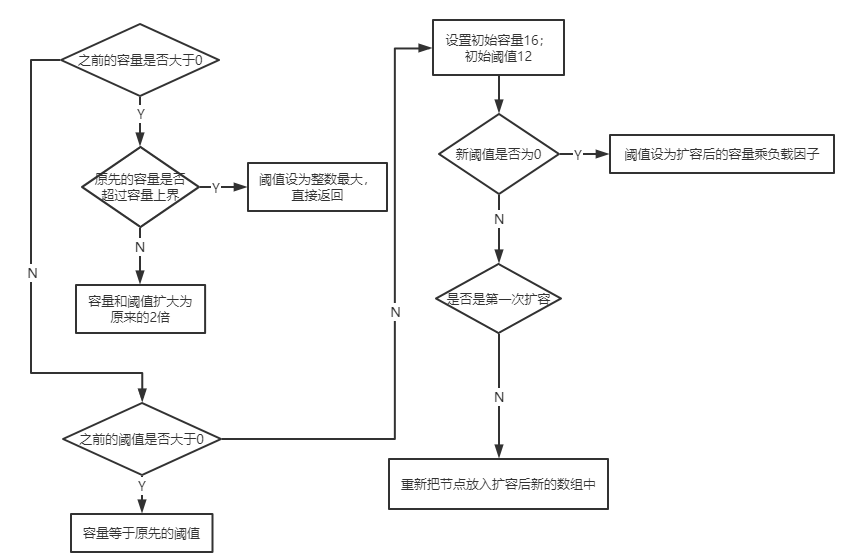``````final Node<K,V>[] resize() {
Node<K,V>[] oldTab = table;
// 存储old table 的大小
int oldCap = (oldTab == null) ? 0 : oldTab.length;
// 存储扩容阈值
int oldThr = threshold;
int newCap, newThr = 0;
if (oldCap > 0) {
// 如果old table数据已达最大，那么threshold也被设置成最大
if (oldCap >= MAXIMUM_CAPACITY) {
threshold = Integer.MAX_VALUE;
return oldTab;
}
// 左移扩大二倍,
else if ((newCap = oldCap << 1) < MAXIMUM_CAPACITY &&
oldCap >= DEFAULT_INITIAL_CAPACITY)
// 扩容成原来二倍
newThr = oldThr << 1; // double threshold
}
// 如果oldThr > 0
else if (oldThr > 0) // initial capacity was placed in threshold
newCap = oldThr;
// 如果old table <= 0 并且 存储的阈值 <= 0
else {               // zero initial threshold signifies using defaults
newCap = DEFAULT_INITIAL_CAPACITY;
}
// 如果扩充阈值为0
if (newThr == 0) {
// 扩容阈值为 初始容量*负载因子
float ft = (float)newCap * loadFactor;
newThr = (newCap < MAXIMUM_CAPACITY && ft < (float)MAXIMUM_CAPACITY ?
(int)ft : Integer.MAX_VALUE);
}
// 重新给负载因子赋值
threshold = newThr;
// 获取扩容后的数组
@SuppressWarnings({"rawtypes","unchecked"})
Node<K,V>[] newTab = (Node<K,V>[])new Node[newCap];
table = newTab;
// 如果第一次进行table 初始化不会走下面的代码
// 扩容之后需要重新把节点放在新扩容的数组中
if (oldTab != null) {
for (int j = 0; j < oldCap; ++j) {
Node<K,V> e;
if ((e = oldTab[j]) != null) {
oldTab[j] = null;
if (e.next == null)
newTab[e.hash & (newCap - 1)] = e;
else if (e instanceof TreeNode)
// 重新映射时，需要对红黑树进行拆分
((TreeNode<K,V>)e).split(this, newTab, j, oldCap);
else { // preserve order
Node<K,V> loHead = null, loTail = null;
Node<K,V> hiHead = null, hiTail = null;
Node<K,V> next;
// 遍历链表，并将链表节点按原顺序进行分组
do {
next = e.next;
if ((e.hash & oldCap) == 0) {
if (loTail == null)
else
loTail.next = e;
loTail = e;
}
else {
if (hiTail == null)
else
hiTail.next = e;
hiTail = e;
}
} while ((e = next) != null);
// 将分组后的链表映射到新桶中
if (loTail != null) {
loTail.next = null;
}
if (hiTail != null) {
hiTail.next = null;
}
}
}
}
}
return newTab;
}``````

1. 判断 HashMap 中的数组的长度，也就是 (Node<K,V>[])oldTab.length() ，再判断数组的长度是否比最大的的长度也就是 2^30 次幂要大，大的话直接取最大长度，否则利用位运算扩容为原来的两倍
``````if (oldCap > 0) {
// 如果old table数据已达最大，那么threshold也被设置成最大
if (oldCap >= MAXIMUM_CAPACITY) {
threshold = Integer.MAX_VALUE;
return oldTab;
}
// 左移扩大二倍,
else if ((newCap = oldCap << 1) < MAXIMUM_CAPACITY &&
oldCap >= DEFAULT_INITIAL_CAPACITY)
// 扩容成原来二倍
newThr = oldThr << 1; // double threshold
}``````

2. 如果数组长度不大于0 ，再判断扩容阈值 threshold 是否大于 0 ，也就是看有无外部指定的扩容阈值，若有则使用，因为 HashMap 中的每个构造方法都会调用 HashMap(initCapacity,loadFactor) 这个构造方法，所以如果没有外部指定 initialCapacity时，初始容量是 16，然后根据 this.threshold = tableSizeFor(initialCapacity); 求得 threshold 的值。

``````// 如果oldThr > 0
else if (oldThr > 0) // initial capacity was placed in threshold
newCap = oldThr;``````

3. 否则，直接使用默认的初始容量和扩容阈值（在 table 刚刚初始化的时候执行）

``````// 如果old table <= 0 并且 存储的阈值 <= 0
else {               // zero initial threshold signifies using defaults
newCap = DEFAULT_INITIAL_CAPACITY;
}``````

• 循环桶中的每个 Node 节点，判断 Node[i] 是否为空，为空直接返回，不为空则遍历桶数组，并将键值对映射到新的桶数组中。
• 如果不为空，再判断是否是树形结构，如果是树形结构则按照树形结构进行拆分，拆分方法在 split 方法中。
• 如果不是树形结构，则遍历链表，并将链表节点按原顺序进行分组。
``````if (oldTab != null) {
for (int j = 0; j < oldCap; ++j) {
Node<K,V> e;
if ((e = oldTab[j]) != null) {
oldTab[j] = null;
if (e.next == null)
newTab[e.hash & (newCap - 1)] = e;
else if (e instanceof TreeNode)
// 重新映射时，需要对红黑树进行拆分
((TreeNode<K,V>)e).split(this, newTab, j, oldCap);
else { // preserve order
Node<K,V> loHead = null, loTail = null;
Node<K,V> hiHead = null, hiTail = null;
Node<K,V> next;
// 遍历链表，并将链表节点按原顺序进行分组
do {
next = e.next;
if ((e.hash & oldCap) == 0) {
if (loTail == null)
else
loTail.next = e;
loTail = e;
}
else {
if (hiTail == null)
else
hiTail.next = e;
hiTail = e;
}
} while ((e = next) != null);
// 将分组后的链表映射到新桶中
if (loTail != null) {
loTail.next = null;
}
if (hiTail != null) {
hiTail.next = null;
}
}``````

## 2.9 get全过程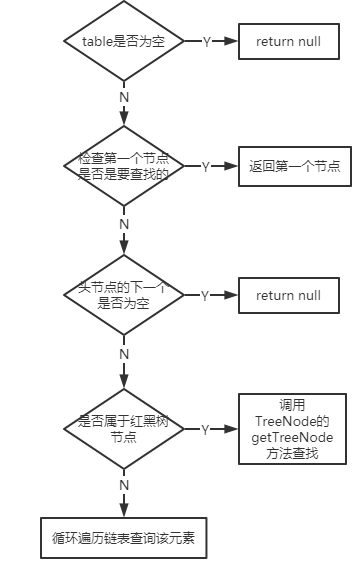``````public V get(Object key) {
Node<K,V> e;
return (e = getNode(hash(key), key)) == null ? null : e.value;
}

final Node<K,V> getNode(int hash, Object key) {
Node<K,V>[] tab; Node<K,V> first, e; int n; K k;

// 找到真实的元素位置
if ((tab = table) != null && (n = tab.length) > 0 &&
(first = tab[(n - 1) & hash]) != null) {
// 总是会check 一下第一个元素
if (first.hash == hash && // always check first node
((k = first.key) == key || (key != null && key.equals(k))))
return first;

// 如果不是第一个元素，并且下一个元素不是空的
if ((e = first.next) != null) {

// 判断是否属于 TreeNode，如果是 TreeNode 实例，直接从 TreeNode.getTreeNode 取
if (first instanceof TreeNode)
return ((TreeNode<K,V>)first).getTreeNode(hash, key);

// 如果还不是 TreeNode 实例，就直接循环数组元素，直到找到指定元素位置
do {
if (e.hash == hash &&
((k = e.key) == key || (key != null && key.equals(k))))
return e;
} while ((e = e.next) != null);
}
}
return null;
}``````

### 3.2 你知道 hash 的实现吗？为什么要这样实现？

JDK 1.8 中，是通过 hashCode() 的高 16 位异或低 16 位实现的：(h = k.hashCode()) ^ (h >>> 16)，主要是从速度，功效和质量来考虑的，减少系统的开销，也不会造成因为高位没有参与下标的计算，从而引起的碰撞。

### 3.4 为什么要保证 capacity 是2的次幂呢？

key在tab中索引位置是由（n-1）&hash计算得到的。

• &运算速度快，至少比%取模运算块
• 能保证 索引值 肯定在 capacity 中，不会超出数组长度
• (n - 1) & hash，当n为2次幂时，会满足一个公式：(n - 1) & hash = hash % n

### 3.5 capacity 都是 2 次幂，如果我们指定 initialCapacity 不为 2次幂时，是不是就破坏了这个规则？

``````/**
* Returns a power of two size for the given target capacity.
*/
static final int tableSizeFor(int cap) {
//cap-1后，n的二进制最右一位肯定和cap的最右一位不同，即一个为0，一个为1，例如cap=17（00010001），n=cap-1=16（00010000）
int n = cap - 1;
//n = (00010000 | 00001000) = 00011000
n |= n >>> 1;
//n = (00011000 | 00000110) = 00011110
n |= n >>> 2;
//n = (00011110 | 00000001) = 00011111
n |= n >>> 4;
//n = (00011111 | 00000000) = 00011111
n |= n >>> 8;
//n = (00011111 | 00000000) = 00011111
n |= n >>> 16;
//n = 00011111 = 31
//n = 31 + 1 = 32, 即最终的cap = 32 = 2 的 (n=5)次方
return (n < 0) ? 1 : (n >= MAXIMUM_CAPACITY) ? MAXIMUM_CAPACITY : n + 1;
}``````

### 3.6 链表法导致链表过深问题为什么不用二叉查找树代替，而选择红黑树？为什么不一直使用红黑树？

LinkedHashMap 保存了记录的插入顺序，在用 Iterator 遍历时，先取到的记录肯定是先插入的；遍历比 HashMap 慢；

TreeMap 实现 SortMap 接口，能够把它保存的记录根据键排序（默认按键值升序排序，也可以指定排序的比较器）

### 3.8 HashMap & TreeMap & LinkedHashMap 使用场景？

HashMap：在 Map 中插入、删除和定位元素时；

TreeMap：在需要按自然顺序或自定义顺序遍历键的情况下；

### 3.9 HashMap 和 HashTable 有什么区别？

1 HashMap 是线程不安全的，HashTable 是线程安全的；

2 由于线程安全，所以 HashTable 的效率比不上 HashMap；

3 HashMap最多只允许一条记录的键为null，允许多条记录的值为null，而 HashTable不允许；

4 HashMap 默认初始化数组的大小为16，HashTable 为 11，前者扩容时，扩大两倍，后者扩大两倍+1；

5 HashMap 需要重新计算 hash 值，而 HashTable 直接使用对象的 hashCode

### 3.10 ava 中的另一个线程安全的与 HashMap 极其类似的类是什么？同样是线程安全，它与 HashTable 在线程同步上有什么不同？

ConcurrentHashMap 类（是 Java并发包 java.util.concurrent 中提供的一个线程安全且高效的 HashMap 实现）。

HashTable 是使用 synchronize 关键字加锁的原理（就是对对象加锁）；

ConcurrentHashMap：

• JDK 1.7 中使用分段锁（ReentrantLock + Segment + HashEntry），相当于把一个 HashMap 分成多个段，每段分配一把锁，这样支持多线程访问。锁粒度：基于 Segment，包含多个 HashEntry。
• JDK 1.8 中使用 CAS + synchronized + Node + 红黑树。锁粒度：Node（首结点）（实现 Map.Entry）。锁粒度降低了。

### 3.11 针对 ConcurrentHashMap 锁机制具体分析（JDK 1.7 VS JDK 1.8）？

JDK 1.7 中，采用分段锁的机制，实现并发的更新操作，底层采用数组+链表的存储结构，包括两个核心静态内部类 Segment 和 HashEntry。

1 Segment 继承 ReentrantLock（重入锁） 用来充当锁的角色，每个 Segment 对象守护每个散列映射表的若干个桶；

2 HashEntry 用来封装映射表的键-值对；

3 每个桶是由若干个 HashEntry 对象链接起来的链表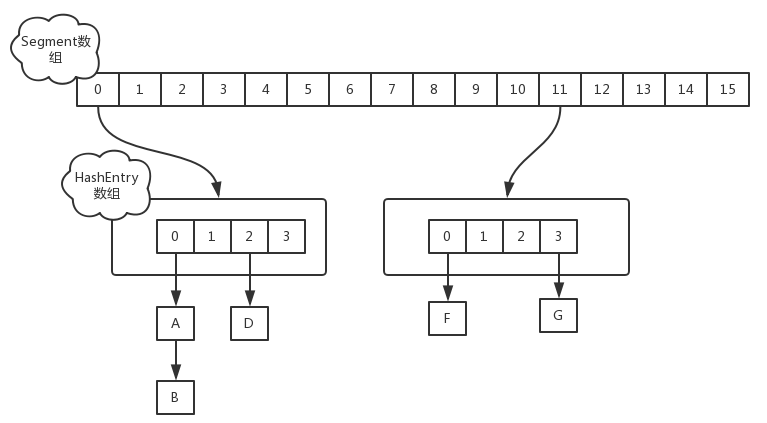JDK 1.8 中，采用Node + CAS + Synchronized来保证并发安全。取消类 Segment，直接用 table 数组存储键值对；当 HashEntry 对象组成的链表长度超过 TREEIFY_THRESHOLD 时，链表转换为红黑树，提升性能。底层变更为数组 + 链表 + 红黑树。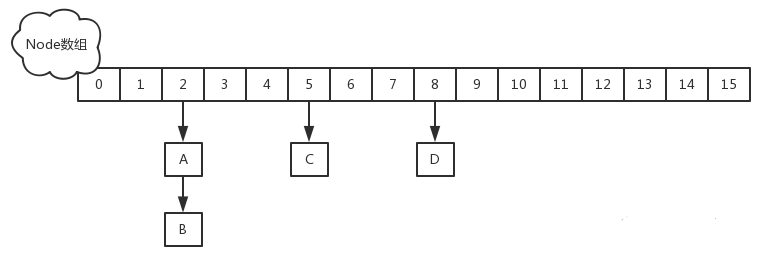### 3.12 ConcurrentHashMap 在 JDK 1.8 中，为什么要使用内置锁 synchronized 来代替重入锁 ReentrantLock？

1 粒度降低了；

2 JVM 开发团队没有放弃 synchronized，而且基于 JVM 的 synchronized 优化空间更大，更加自然。

3 在大量的数据操作下，对于 JVM 的内存压力，基于 API 的 ReentrantLock 会开销更多的内存。

### 3.14 ConcurrentHashMap 简单介绍

1 重要的常量：

private transient volatile int sizeCtl;

• 当为负数时，-1 表示正在初始化，-N 表示 N - 1 个线程正在进行扩容；
• 当为 0 时，表示 table 还没有初始化；
• 当为其他正数时，表示初始化或者下一次进行扩容的大小。

2 数据结构：

• Node 是存储结构的基本单元，继承 HashMap 中的 Entry，用于存储数据；
• TreeNode 继承 Node，但是数据结构换成了二叉树结构，是红黑树的存储结构，用于红黑树中存储数据；
• TreeBin 是封装 TreeNode 的容器，提供转换红黑树的一些条件和锁的控制。

3 存储对象时（put() 方法）：

1. 如果没有初始化，就调用 initTable() 方法来进行初始化；
2. 如果没有 hash 冲突就直接 CAS 无锁插入；
3. 如果需要扩容，就先进行扩容；
4. 如果存在 hash 冲突，就加锁来保证线程安全，两种情况：一种是链表形式就直接遍历到尾端插入，一种是红黑树就按照红黑树结构插入；
5. 如果该链表的数量大于阀值 8，就要先转换成红黑树的结构

4 扩容方法 transfer()：默认容量为 16，扩容时，容量变为原来的两倍。

• helpTransfer()：调用多个工作线程一起帮助进行扩容，这样的效率就会更高。

5 获取对象时（get()方法）：

• 计算 hash 值，定位到该 table 索引位置，如果是首结点符合就返回；
• 如果遇到扩容时，会调用标记正在扩容结点 ForwardingNode.find() 方法，查找该结点，匹配就返回；
• 以上都不符合的话，就往下遍历结点，匹配就返回，否则最后就返回 null。

-- 展开阅读全文 --

« 上一篇 07-24